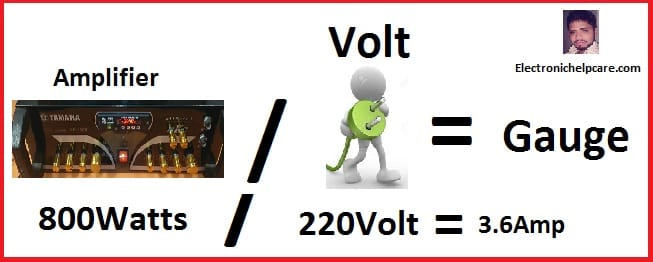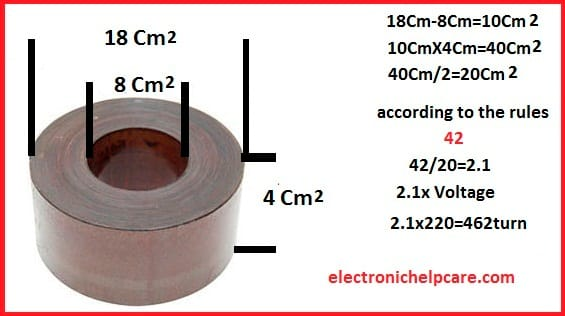# toroidal transformer for amplifier

## toroidal transformer for the amplifier:

If you want to make a toroidal transformer, first you have to decide where you are going to use it. how many watts, voltages, and amperes you need. here in this article, we made a transformer for an amplifier. Before making a must decide, How many watts need? How many amperes need and How many Voltages need?

Let’s watch the video first

First, you have to decide how many watts will be your transformer. before you need to know about the ampere of the wire.

## Gauge of the wire

Gauge………….………………Ampere

7…………………………………44.2

8…………………………………33.3

9…………………………………26.5

10……………….……………….21.2

11………………………….…….16.6

12………………………………….13.5

13………………………………….10.5

14………………………………….8.3

15………………………………….6.6

16………………………………….5.2

17………………………………….4.1

18………………………………….3.2

19………………………………….2.6

20………………………………….2.0

21………………………………….1.6

22………………………………….1.2

23………………………………….1.0

24………………………………….0.8

25………………………………….0.6

26………………………………….0.5

27………………………………….0.4

28………………………………….0.3

29………………………………….0.29

30………………………………….0.22

For the primary winding, you have to calculate the ampere of the wire.

How to calculate the wire ampere?## (Amplifier watts)/(AC voltage)=Ampere.

if your amplifier is 500 Watts and your ac voltage is 220. So 500/220=2.27 Ampere wire you need.

so 19-number gauge wire you have to use for the primary.

Now how many turns need for the primary?

Transformer core area/42=turn per voltage.

For Example, your transformer core area is…….. 8 following the Video. So 8 is your core area. Now Use the rules.

42/8=5.24 is the turn per volt. for one voltage need 5.24 turn. 42 is the rule.

So if you have 110 voltage for the primary. 110 X 5.24=576.4 turn needed. we can give 10 turns extra for the good.

Or for the 220 voltage 220 X 5.24=1152.8 turn needed for the primary.## Secondary wire and ampere calculate

How many amperes do you need?

To calculate how many amperes you need.

here in this video, you can watch how to calculate ampere.

If you use 8 transistors you need…..?

we know per transistor need 1.2 amperes so  8 X 1.2=9.6 ampere needs. 13 gauge number wire we need for the secondary wire.

And Now calculate the secondary wire. we need 35 voltage so 35 X 5.24=183.4 turn needs. we can make it 185 turns for a good performance.

transistor circuit diagram of 2sa1943 and 2sc5200

Thanks a lot to be with us. If you want another post then please visit our website.

We have another post for you. like repairing amplifier,

Please like comment and share our post.